## Distinct Elements in Arithmetic Tables

Balmoral Software

Prominent patterns are evident in arithmetic tables when only distinct entries are shown. This paper describes some interesting results and explanations about these patterns.

### Selection Criterion

Consider the ordinary multiplication table used to teach elementary-school children: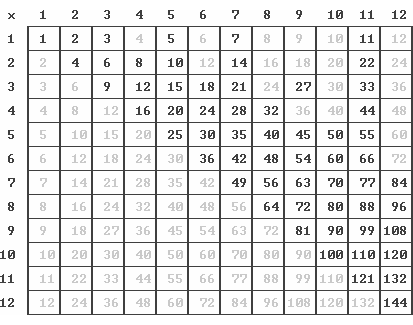This table can be modified in such a way as to include only distinct numbers, shown in darker text above. Duplicate products are excluded from the modified table; for example, the entry of 20 in row 2 and column 10 is not shown since it appears in row 4 and column 5 of a smaller table, closer to the diagonal.

A consistent selection criterion can be established for all arithmetic operators that identifies distinct entries in the associated tables. This criterion consists of picking an entry occurring in the smallest square table, which in most cases is equivalent to selecting entries with row and column indices that are closer together than any others producing the same table entry. For commutative operators, the arithmetic table is symmetric with respect to the main diagonal extending from the upper-left corner to the lower-right one. By convention, entries in the lower-triangular portion of arithmetic tables for commutative operators are excluded from consideration as distinct entries since they already appear in the upper region. Excluding the upper-triangular region instead would produce the same patterns, but with rows and columns transposed.

The selection criterion described above can be applied to table entries in any order, producing the same result.

Let R and C denote the row and column indices in an N x N arithmetic table, R,C,N ∈ ℕ, 1 ≤ R ≤ N, 1 ≤ C ≤ N. The usual notation (R,C) will be used to indicate the entry in row R and column C; with R ≤ C for commutative operators. The following notes describe the selection criteria for distinct entries in each of the arithmetic tables:

Multiplication: A distinct product in the table selected to minimize the associated row and column indices has R and C closer together than any other factor pair having a product equal to RC. Equivalently, R and C are central divisors, as defined below.

Division: A quotient in lowest terms satisfies the selection criterion, since any other fraction with the same value has both numerator and denominator that are larger multiples of those in the lowest-terms formulation, and therefore farther apart. The row and column indices of a distinct table entry have no common factors; that is, their greatest common divisor (gcd) is 1.

Addition: As with the preceding operations, a distinct sum in the addition table selected to minimize the associated row and column indices has R and C closer together than any other pair having the same sum S = R + C. If S is even, then the closest pair of such addends is R = C = S/2, in which case C - R = 0. If S is odd, then we take R = (S - 1)/2 and C = (S + 1)/2, with C - R = 1. The distinct entries form the main diagonal and first superdiagonal of the table.

Subtraction: A distinct entry in the subtraction table is selected to minimize both of the associated row and column indices since the difference between the indices is fixed. This occurs when the row or column index is at its minimum value of 1. As a result, distinct entries occur in the first row and first column of the table.

Summary:

 Selection criterion for distinct entries Operation Multiplication {R,C} are central divisors Division gcd(R,C) = 1 Addition C - R is 0 or 1 Subtraction min{R,C} = 1

### The Multiplication Table

In the display of a large modified multiplication table (MMT), we can let each pixel of a square bitmap image represent a distinct entry in the table. We can ignore the numeric value of each entry and focus solely on whether it is present (dark pixel) or absent (white pixel) in the MMT. Various distinctive patterns are immediately evident; for example, the 600 x 600 MMT is: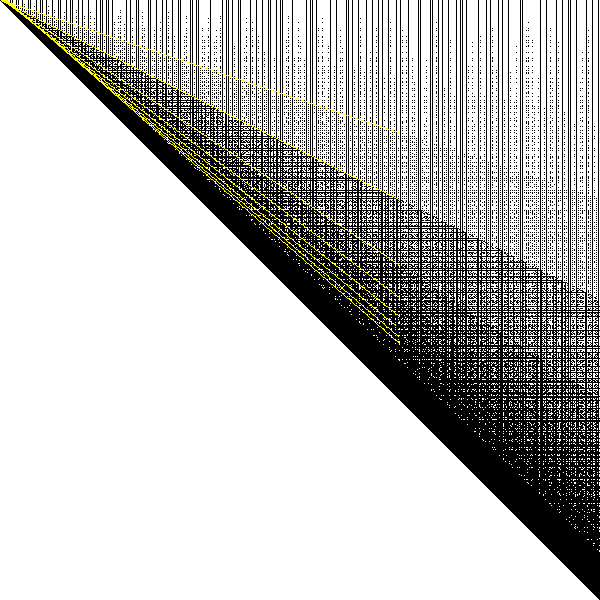We'll explore these patterns in the sections below.

#### Central Divisors

Define the central divisors of a natural number N as the factor pair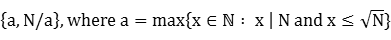As the factor x increases towards its limit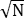, its paired factor N/x decreases towards the same limit. Therefore, the central divisors of N have the property that they are closer together than any other factor pair of N. For example, {18,20} are the central divisors of their product 360. But {6,74} are not the central divisors of 444 since 12 x 37 = 444 and {12,37} are closer together.

The factors a and N/a above are referred to as the lesser central divisor (LCD) and greater central divisor (GCD) of N. Capital letters are always used in this paper for these acronyms to avoid confusion with greatest common divisor (gcd). By convention, the row index is the LCD and the column index the GCD of any product present in the MMT. LCDs and GCDs are listed as OEIS sequences A033676 and A033677, respectively. The frequency distributions of LCDs and GCDs are discussed in a subsequent section.

A factor pair {a,b} is a set independent of the size order of its elements, but we usually follow the convention of listing the smaller factor first when the relative factor sizes are known.

Obviously, {a,b} are always central divisors when a = b. Another result is that {1,p} are central divisors if and only if p = 1 or p is a prime, by Lemma 2 below. Similarly, {p,q} are central divisors when p and q are primes, by Lemma 3. A general result for {n,p} is presented in Lemma 7.

There are areas of the MMT where quantum jumps in the density of distinct entries create "shadow lines" due to the effect of the central divisors of the row and column indices. Let {a,b} be the central divisors of the column index C, with a ≤ b. Since C = ab and
(a - 1)(b - 1) + 1 = (1 - 1/a)ab - a + 2,
then by Lemma 8, any column index with a as its LCD will have an unbroken segment of dark pixels starting at the line
R = (1 - 1/a)C - a + 2
and extending vertically down to the main diagonal R = C. For a ≥ 2, the slopes of these lines are 1/2, 2/3, 3/4, 4/5 and so on, as shown by the yellow annotations in the preceding and following diagrams. In the case of a prime-numbered column, we have a = 1 and the shadow line is horizontal at R = 1, so the MMT will have all of its entries above the diagonal present. These are the full-height vertical black lines in the preceding diagram.

When C is a square, a reaches its maximum value of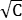and the top of the vertical segment of dark pixels occurs at row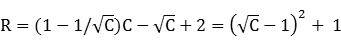By Lemma 8 and Lemma 10, this minimum value of R is greater than or equal to the lower limit for R for non-square values of C, so there is always a solid band of entries in the MMT between the main diagonal and a narrow parabola above it.

Analogously for a row R with central divisors {a,b}, a ≤ b,

(a + 1)(b + 1) - 1 = (1 + 1/a)ab + a,
and any row index R with a as its LCD will have an unbroken segment of dark pixels extending horizontally from the main diagonal and ending at the line
C = (1 + 1/a)R + a,
which can be written
R = [1 - 1/(a + 1)]C - a2/(a + 1)
These lines have the same slopes and nearly the same intercepts as those for the column segments:

 Vertical segments a Slope Intercept 1 0 1 2 1/2 0 3 2/3 -1 4 3/4 -2 5 4/5 -3 ...
 Horizontal segments a Slope Intercept 1 1/2 -1/2 2 2/3 -4/3 3 3/4 -9/4 4 4/5 -16/5 ...
The table entries that have been described in this section are shown below for the 600 x 600 MMT: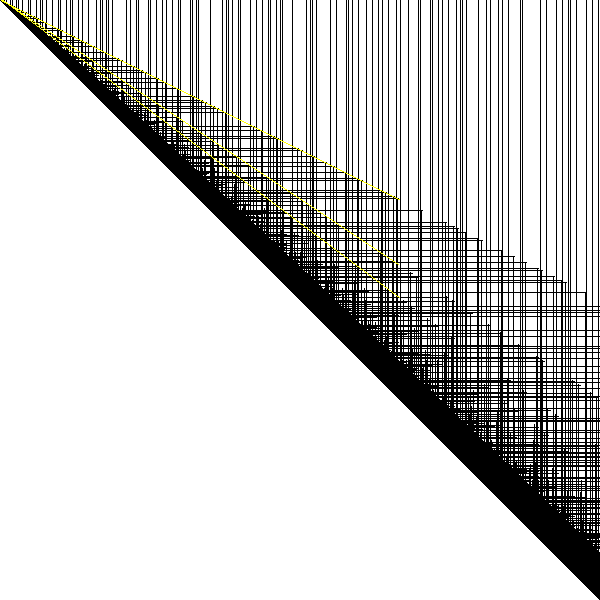#### Blank Regions

Other contributors to shadow lines are the edges of blank regions occurring in the upper parts of the MMT for smaller row indices R. For example, when the column index C is even, all rows in the MMT for which R ≤ C/2 - 1 have blank entries, by Lemma 11 with a = R, b = C and k = 2. This behavior is illustrated by displaying only the even columns of the MMT: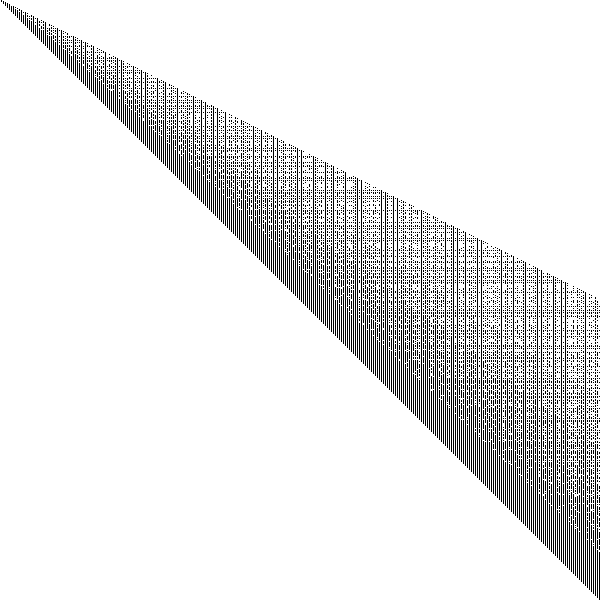Next, we consider columns that are multiples of 3. To exclude the shadow line already considered in the first example, we'll examine columns that are odd multiples of 3 (C mod 6 = 3):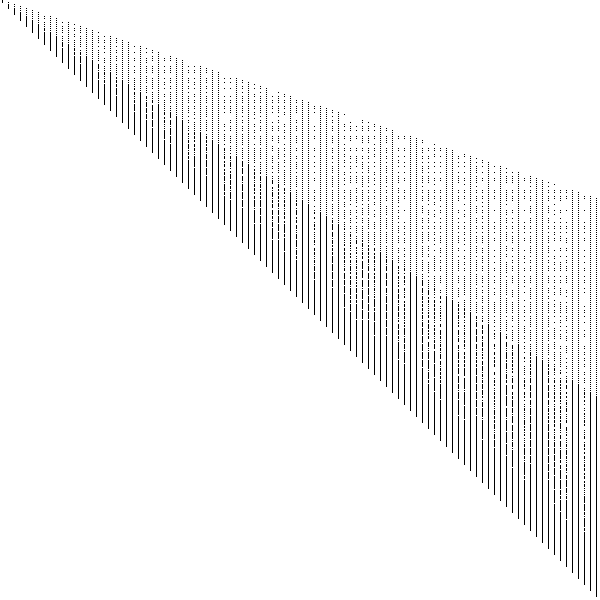Applying Lemma 11 with a = R, b = C and k = 3, all MMT entries are blank in the top third of the table where
R ≤ C/3 - 1
A shadow line is apparent at R = (2/3)C - 1 due to significantly more numerous central divisors in the third of the table closest to the diagonal than in the middle third. For the subset of column indices that are triples of a prime, all entries below the shadow line are central divisors, and exactly half of the entries above the line:Proof. Let C = 3p for a prime p and consider the lowest third of the MMT:
2C/3 - 1 ≤ R ≤ C

2p - 1 ≤ R ≤ 3p

Since {3,p} are central divisors by Lemma 3 and the above inequality is contained within
2p - 1 ≤ R ≤ 4p + 3,
then the conditions of Lemma 8 are met with a = 3, b = p and m = R, and so {R,3p} are central divisors.

In the adjacent middle third of the table, we have

C/3 ≤ R ≤ 2C/3 - 2

p ≤ R ≤ 2p - 2

By Lemma 15, {R,3p} are central divisors if and only if R is odd, QED.

Additional shadow lines can be identified in a similar manner for other divisors of C, but they become increasingly faint since more columns are excluded in order to mask the shadow lines from previous selections.

The entries identified in both sections above account for more than 90% of the entries in the MMT and its most significant patterns: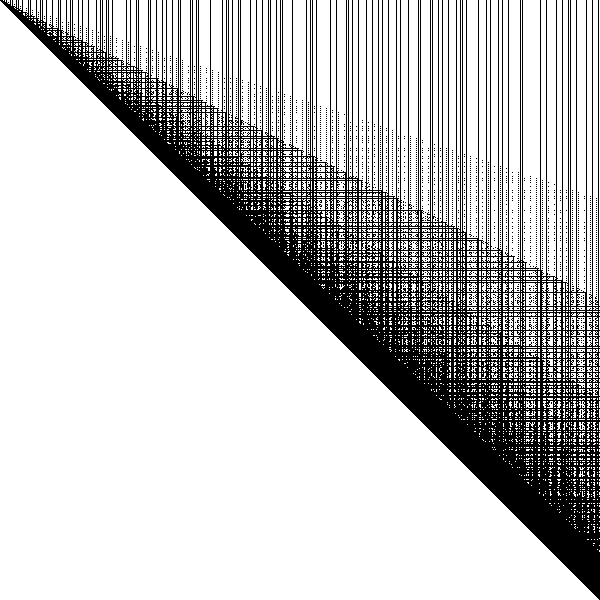#### Limiting Behavior of the Fraction of Distinct Products

Even though entire table columns occasionally appear due to primes, trends toward sparseness continue in larger MMTs, and it has been shown that the fraction of distinct entries in the table approaches zero as the table size grows to infinity.

Let F(N) denote the number of entries in the N x N MMT (tabulated as OEIS sequence A027424). Then

H(N) = F(N)/N2
is the fraction of table entries that are distinct. The first few values of F(N) and H(N) are:
 F(1) = 1 H(1) = 1 F(2) = 3 H(2) = 3/4 F(3) = 6 H(3) = 2/3 F(4) = 9 H(4) = 9/16
The mathematician Paul Erdős proved in 1955 [1,2] that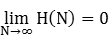The convergence is relatively slow, as can be seen in the chart below. Due to the influence of primes, H has a locally non-monotonic behavior:A result by Ford  shows that H(N) is bounded by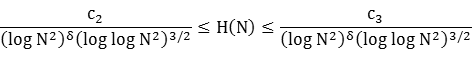for N ≥ 3, where δ = 1 - (1 + log log 2)/log 2 = 0.08607. These bounds are shown in the preceding diagram with c2 = 0.8 and c3 = 1.5 for N ≥ 30.

### The Division Table

An analogous modification can be made for the N x N division table, wherein the only quotients shown are the ones in lowest terms. The distinct entries in the 9 x 9 modified division table (MDT) are in darker text below:Only quotients created from relatively-prime numerator and denominator are shown in the MDT, equivalent to them having a greatest common divisor (gcd) of 1. As a result, there is an obvious symmetry in the table with respect to the main diagonal. Extending the MDT to larger sizes produces a repetitious complexity. Again representing table entries by dark pixels, the 600 x 600 MDT has a lace-like appearance, with diagonal and rectilinear patterns evident: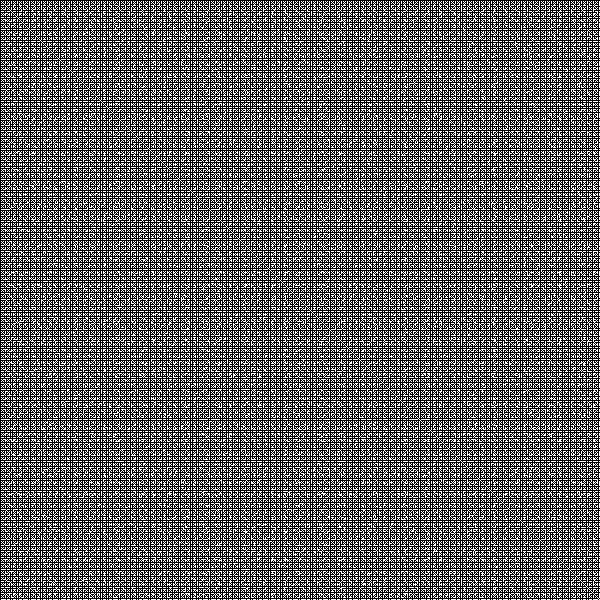This is a plan view of Euclid's orchard.

In the remainder of this paper, we define a proper divisor k of a composite natural number n as one that is neither 1 nor n: 2 ≤ k ≤ n/2.

#### First Row and Column

Since gcd(n,1) = 1 for any natural number n, all entries in row 1 and all entries in column 1 are present in the MDT.

#### Prime-numbered Rows and Columns

Since primes are coprime to all natural numbers other than their own multiples, entries in prime-numbered rows and columns are all present except when the row index is a multiple of the column index or vice-versa.

Entries described in the preceding two sections are shown below for the 600 x 600 MDT: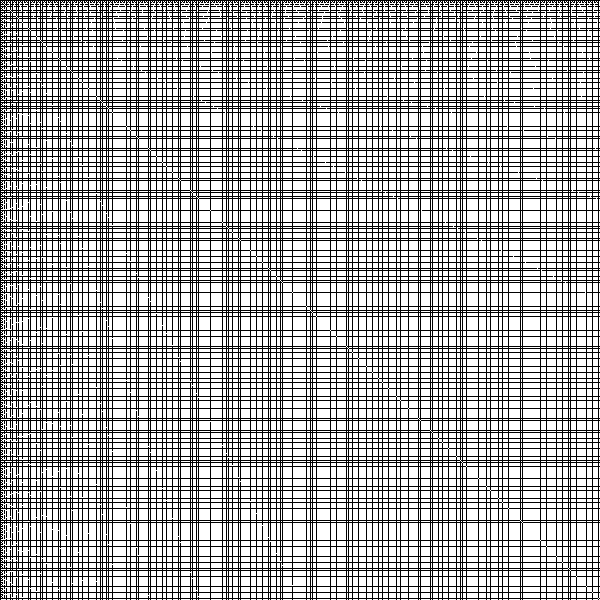#### Ascending Diagonals

Ascending diagonals associated with primes are included in the MDT. Assume R + C = p for some prime p ≤ 2N. We have C = p - R ≥ 1, so 2 ≤ R + 1 ≤ p, or 1 ≤ R < p. Then the conditions of Lemma 16 apply for n = R, so R and C are coprime and therefore (R,C) appears in the MDT.

The points (R,C) form ascending diagonals of included entries over the entire MDT. As p increases from 2 to N, then R < p and the ascending diagonals transition from the upper-left corner to the main antidiagonal connecting the lower-left and upper-right corners. As p further increases from N + 1 to 2N, then R < p - N and the ascending diagonals transition from the main antidiagonal to the lower-right corner.

#### Descending Diagonals

If R = C, then all entries on the main diagonal except (1,1) are excluded from the MDT since their quotients are 1.

If |R - C| = 1, then the entry R/C = R/(R ± 1) is in lowest terms since the numerator and denominator are consecutive integers and therefore coprime. As a result, all entries on the two diagonals adjacent to the main diagonal are included.

If |R - C| is a prime p, then p < N and the table entry is R/C = R/(R ± p), subject to 1 ≤ R ± p ≤ N. If k is a proper divisor of R and R is not a multiple of p, then the entry can be written as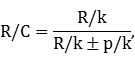which cannot be a quotient of integers since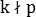. Therefore, R/C is in lowest terms. However, if R = mp for some natural number m, then the entry can be written as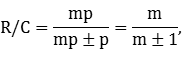so the table entry is not in lowest terms. Therefore, the entries on prime-numbered descending diagonals are included, except at intervals of p.

The entries described in the preceding two sections are shown below for the 600 x 600 MDT: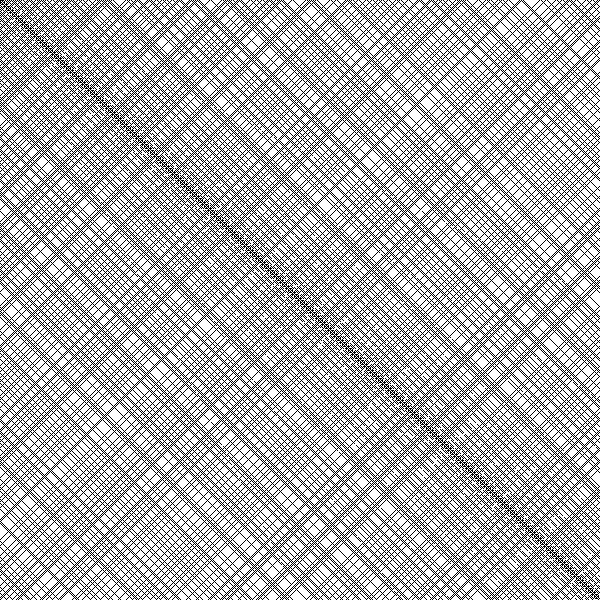The behavior of descending diagonals accounts for the fuzziness adjacent to the main diagonal, where first every table entry is present (|R - C| = 1), then half of them (|R - C| = 2), then two-thirds of them (|R - C| = 3), then four-fifths of them, and so on. The detail can be seen using various colors in a smaller (30 x 30) MDT: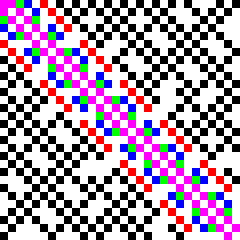Entries classified in all of the previous sections are shown here for the 600 x 600 MDT, and account for most of the entries present in the table: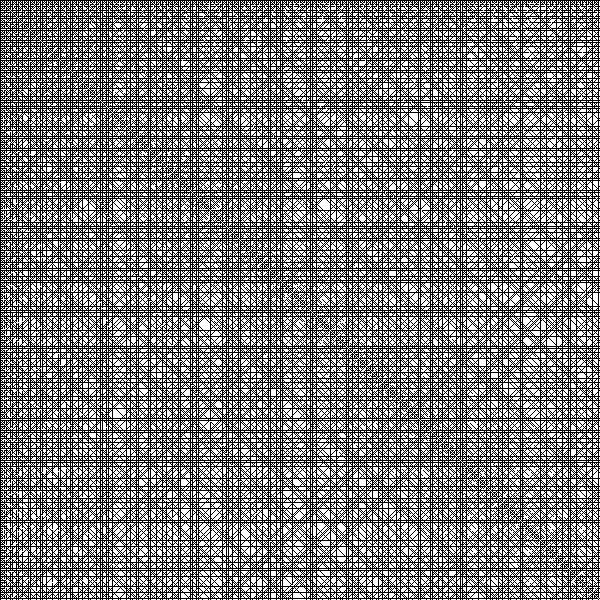#### Limiting Behavior of the Fraction of Distinct Quotients

The fraction of distinct entries in the MDT approaches 6/π2 = 60.8% as the table size grows to infinity. This limit is the probability of a quotient of random integers being in lowest terms [4,5].

Let F(N) denote the number of distinct entries in the N x N MDT. Equivalently, F(N) is the number of lowest-terms quotients of the natural numbers 1,...,N, with F(1) = 1. For N ≥ 2, the count of new entries in the last row and column of the N x N MDT is F(N) - F(N - 1), which is the number of lowest-terms equivalents of quotients of the form x/N or N/x, where 1 ≤ x ≤ N. The Euler totient function φ(N) (OEIS A000010) defines the quantity of natural numbers up to N that are relatively prime to N, so

F(N) - F(N-1) = 2φ(N),
since each lowest-terms fraction appears in the MDT along with its reciprocal. The solution to this difference equation is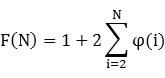(1)
As before, the fraction of distinct table entries is
H(N) = F(N)/N2
The first few values of F(N) and H(N) are:
 F(1) = 1 H(1) = 1 F(2) = 3 H(2) = 3/4 F(3) = 7 H(3) = 7/9 F(4) = 11 H(4) = 11/16
From , we have φ(1) = 1 and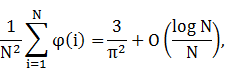(2)
so from (1) and (2),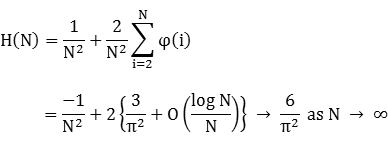The convergence of H(N) to its limit is considerably faster for the MDT than in the MMT. Due to relatively larger values of φ(N) for prime N, H(N) has a locally non-monotonic behavior: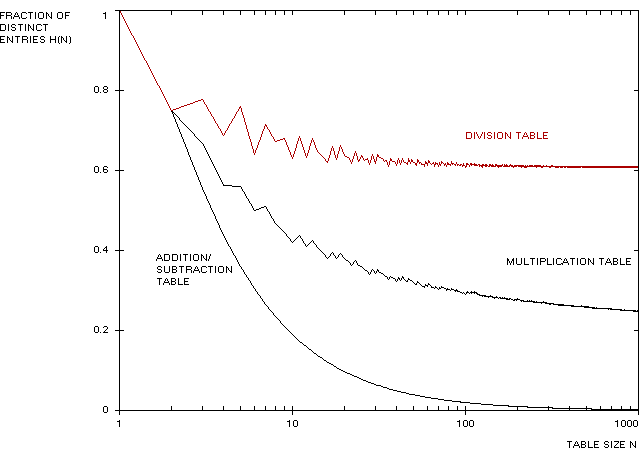Numerical values of H(N) may be computed for small N using an application of a gcd algorithm for φ(i) :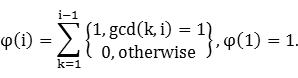If storage is available for all primes p ≤ N, computation of φ for larger N is much faster using Euler's product formula: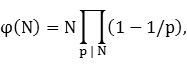(3)
where the product is taken over all primes p dividing N. This formula can be implemented iteratively with the following steps:
input: N
φ ← N
for all primes p ≤ N do
if p | N, then φ ← (φ/p)(p - 1)
output: φ

### The Addition and Subtraction Tables

A modified addition table constructed in the same way as the preceding tables has distinct entries on the main diagonal and first superdiagonal only: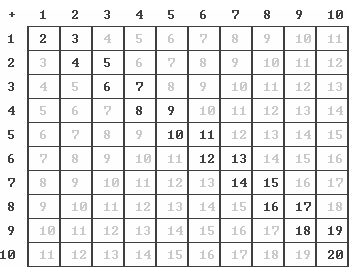A modified subtraction table where the row is subtracted from the column has distinct entries in the first row and first column only: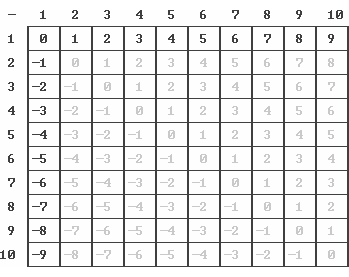In both cases, the modified N x N table has F(N) = 2N - 1 entries, and the fraction of distinct entries converges to zero: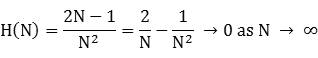Since the modified addition and subtraction tables are not affected by primes, the H(N) function is smooth: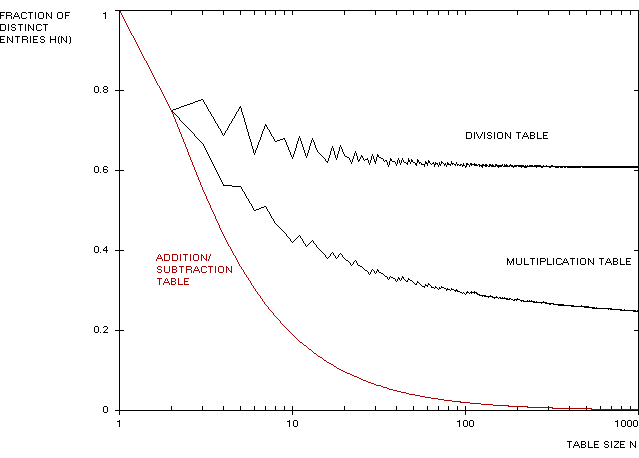### The Totient Function

When plotted versus its argument on an inverted vertical scale like in the arithmetic tables above, the totient function itself exhibits shadow lines similar to those in the MMT: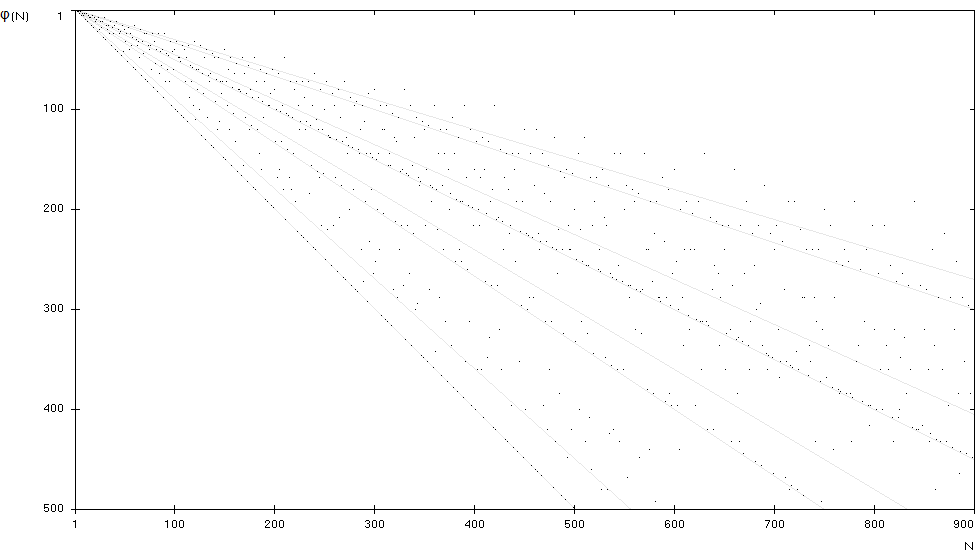These shadow lines occur because in (3), the relative value φ(N)/N is frequently dominated by the largest factors in the product, which are associated with powers of the smallest primes 2 or 3 in the prime factorization of N. For example, if N is even but not a multiple of 3, then φ(N)/N is less than or equal to (and often near) the value (1 - 1/2) = 1/2. Similarly, if N is an odd multiple of 3, then φ(N)/N is less than or equal to (and often near) the value (1 - 1/3) = 2/3. The pattern continues if N is a multiple of 6, whence φ(N)/N is less than or equal to (and often near) the value (1 - 1/2)(1 - 1/3) = 1/3. Since φ(N) = N - 1 for prime N, there are also values of φ(N)/N close to 1. Roughly 2/3 of the values of φ(N) occur within 10% of one of these four shadow lines, indicated by the paired boundary lines in the diagram above for N ≤ 900. The actual counts are:
 Near N/3 line: 102 11% Near N/2 line: 188 21% Near 2N/3 line: 96 11% Near N-1 line: 182 20% Remainder: 332 37%
These proportions remain consistent for larger values of N.

### Frequency Distribution of Central Divisors

We turn now to the distribution of LCDs (row numbers) of the distinct entries in the multiplication table. Subsequent sections discuss the frequency distributions of LCDs in the general case where the paired GCD is not constrained, and of GCDs in the MMT.

#### Lesser Central Divisor (Modified Multiplication Table)

Let fN(a) denote the number of occurrences of a as the LCD of the entries in the N x N MMT; it follows that a ranges from 1 to N and fN(a) is the number of entries in row a of the MMT. For example, by Lemma 2 the central divisors comprising fN(1) are {1,1} and {1,p} for all primes p in the range 2,...,N. We also know that:
{2,p} are central divisors for all primes p, 2 ≤ p ≤ N, by Lemma 3
{2,4} are central divisors by inspection
{2,c} are not central divisors for all composite c, 6 ≤ c ≤ N by Lemma 17 with n = 2
Therefore,
fN(1) = fN(2) = π(N) + 1,
where π is the prime counting function. In general, the central divisors counted in fN(a) are {a,n}, where
 a ≤ n ≤ N (4)
The left inequality ensures that a is the lesser of the central divisors {a,n}, and the right inequality that both divisors are within the range found in the MMT. A histogram of f500(a) is shown below, along with upper and lower bounds defined in the following sections: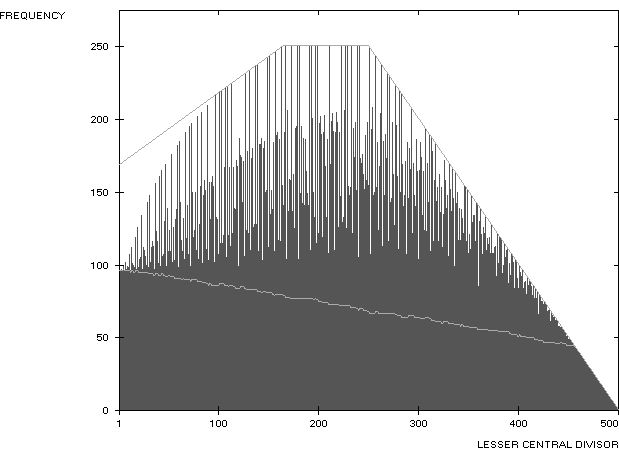This frequency distribution has the same general shape for all values of N.

Tail Values

When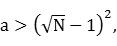the exact value of fN(a) is
fN(a) = N - a + 1
This corresponds to the solid band of entries in the MMT adjacent to the main diagonal.

Proof. We have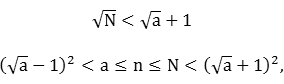so all values of n satisfying (4) are within the range (20) of Lemma 13, and thus correspond to central divisors, QED.

Now assume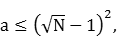so that the range of n covers two intervals: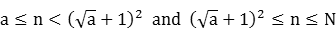The first interval can be written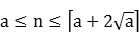and contains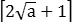values of n, all of which are central divisors by Lemma 13.

The second interval can be written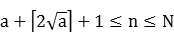We consider only prime values of n in this interval to establish a lower bound for fN(a). Additional central divisors may exist for composite n. Since a ≤ n < 2n + 1, the conditions of Lemma 7 are satisfied and {a,n} are central divisors for all prime n. The number of primes in the second interval is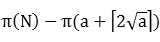Including both intervals, a lower bound for fN(a) thus is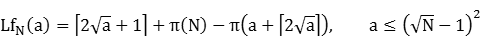A list of lower bound values for N = 500 is tabulated here.

Upper Bounds

Tight upper bounds for fN(a) can be piecewise defined based on known characteristics of sequences of central divisors having the same LCD. Not surprisingly, these upper bounds are established by local maxima occurring at prime LCD values. In fact, most of the upper bounds described here are exact values of fN(p) for prime p. The values of fN(1) and fN(2) were determined above, so we henceforth assume p ≥ 3.

If a is a prime and n complies with the limits in Lemma 7, then {a,n} are central divisors. However, if a is composite and n is subject to the same limits, there often can exist rational numbers r such that 1 < r2 ≤ n/a and both ra and n/r are integers. It follows that {ra,n/r} are closer together than {a,n} and therefore fN(a) excludes the count for the factor pair {a,n}. An example is the factor pair {102,160} that are not central divisors (r = 20/17), but would be if a = 102 was treated as a prime.

Consider the factor pair {p,n} for a prime p ≥ 3. Since n cannot exceed N in the MMT, then by Lemma 7, {p,n} are central divisors for all primes p ≤ N and all values of n in the range

 p ≤ n ≤ min{2p,N}, p ≤ N (5)
The value n = 2p + 1 is excluded so that the interval (5) will juxtapose subsequent intervals described below. Applying Lemma 18 with i = p, j = min{2p,N}, k = 1, m = 0, the quantity of values of n in (5) is min{p,N - p} + 1.

For p < N/2, we have 2p + 1 ≤ N and by Lemma 20, {n,p} are central divisors for odd values of n in the range

 2p + 1 ≤ odd n ≤ min{3p,N}, p < N/2 (6)
Applying Lemma 18 with i = 2p + 1, j = min{3p,N}, k = 2, m = 1, the quantity of values of n in (6) is ⌊ min{p + 1,N - 2p + 1}/2 ⌋.

For p < N/3, we have 3p + 1 ≤ N and by Lemma 21, {n,p} are central divisors for values of n such that

3p + 1 ≤ n ≤ min{5p,N} and n mod 6 = ±1
If p ≥ N/5, then min{5p,N} = N. But if p < N/5 and we continue to use N as the maximum value of n (rather than the smaller 5p), we are overcounting the values of n to provide an upper bound for fN(p). This approach is used for simplicity since the exact range for n when p < N/5 involves a complex selection of 8 values out of every 30. Similar remarks hold for p < N/q for primes q ≥ 7. Therefore, we have
3p + 1 ≤ n ≤ N when n mod 6 = 1, p < N/3
 and (7)
3p + 1 ≤ n ≤ N when n mod 6 = 5, p < N/3
Applying Lemma 18 twice with i = 3p + 1, j = N, k = 6, m = 1 and 5, the quantity of values of n in (7) is
⌊ (N - 1)/6 ⌋ - ⌈ p/2 ⌉ + ⌊ (N - 5)/6 ⌋ - ⌈ (3p - 4)/6 ⌉ + 2

= 2⌊ N/6 ⌋ + ⌊ [(N mod 6) + 3]/4 ⌋ - p

The results (5), (6) and (7) cover adjacent intervals for n over its full range of possible values, and can be summarized as follows:
 Range of p Range(s) of n Quantity of n values p ≥ N/2 p ≤ n ≤ N (5) N - p + 1 N/3 ≤ p < N/2 p ≤ n ≤ 2p (5) p + 1 2p + 1 ≤ odd n ≤ N (6) ⌊ (N + 1)/2 ⌋ - p p < N/3 p ≤ n ≤ 2p (5) p + 1 2p + 1 ≤ odd n ≤ 3p (6) (p + 1)/2 3p + 1 ≤ n ≤ N & n mod 6 = ±1 (7) 2 ⌊ N/6 ⌋ + ⌊ [(N mod 6) + 3]/4 ⌋ - p
Summing the number of values in each range, we have the following piecewise definition for an upper bound UfN(a) of fN(a) for all a:
 Range of a UfN(a) Description a ≥ N/2 N - a + 1 Line of slope -1 N/3 ≤ a < N/2 ⌊ (N + 3)/2 ⌋ Horizontal line a < N/3 ⌊ (a + 3)/2 ⌋ + 2 ⌊ N/6 ⌋ + ⌊ [(N mod 6) + 3]/4 ⌋ Line of slope 1/2
Since {N,N} is the only factor pair when a = N, we have fN(N) = 1. From right to left, the consecutive points of the polygonal upper bound are:
UfN(N) = 1

UfN(⌈ (N - 1)/2 ⌉) = ⌊ (N + 3)/2 ⌋

UfN(2 ⌊ (N + 3)/2 ⌋ - 4 ⌊ N/6 ⌋ - 2 ⌊ [(N mod 6) + 3]/4 ⌋ - 3) = ⌊ (N + 3)/2 ⌋

UfN(1) = 2 + 2 ⌊ N/6 ⌋ + ⌊ [(N mod 6) + 3]/4 ⌋

For example, at N = 500 the points are:
Uf500(500) = 1

Uf500(250) = 251

Uf500(165) = 251

Uf500(1) = 169

#### Lesser Central Divisor (General Case)

Let gN(a) denote the number of occurrences of a as the LCD of all of the natural numbers 1,...,N2, rather than just the subset of those numbers occurring in the N x N MMT. As before, a ranges from 1 to N, but we now have a larger maximum for n:
 a ≤ n ≤ N2/a, (8)
so that the product an is within range. For example, the central divisors comprising gN(1) are {1,1} and {1,p} for all primes p in the range 2,...,N2, by Lemma 2. It follows that
gN(1) = π(N2) + 1
A histogram of g500(a) is shown below, along with upper and lower bounds defined in the following sections: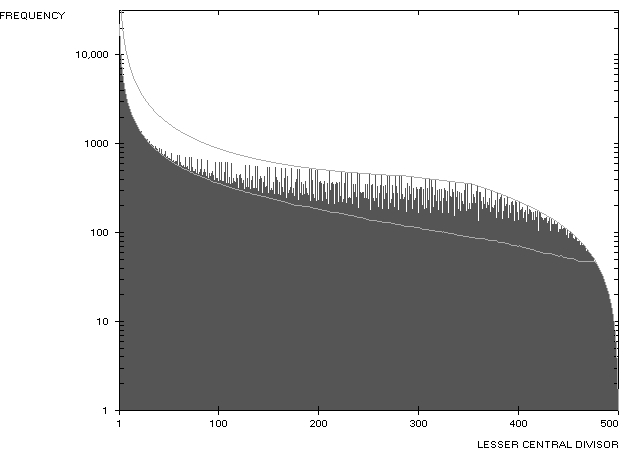Tail Values

When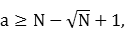(9)
the exact value of gN(a) is
gN(a) = 2(N - a) + 1
Proof. We have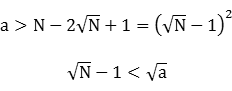(10)
Rewriting (9),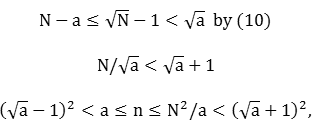so all values of n satisfying (8) are within the range (20) of Lemma 13, and thus correspond to central divisors. The number of values of n in (8) is
 ⌊ N2/a ⌋ - a + 1 (11)
From (9),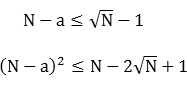Adding both sides of the previous two inequalities, we have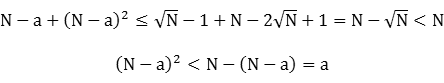(12)
We can write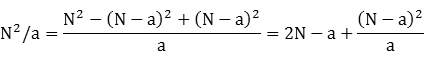By (12), the fractional portion is between 0 and 1, so
⌊ N2/a ⌋ = 2N - a
From (11), we have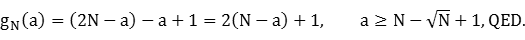Working downwards from a = N, gN takes on increasing odd values until the limit (9) is reached.

Lower Bound

Now assume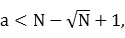so that the range of n covers two intervals as in the preceding section. The results in that section apply with N replaced by ⌊ N2/a ⌋, so a lower bound for gN(a) is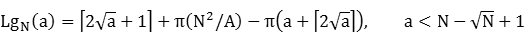Upper Bounds

Substituting ⌊ N2/a ⌋ for N in the table above, we have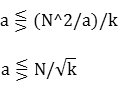Range of a UgN(a)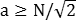⌊ N2/a ⌋ - a + 1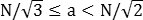⌊ (N2/a + 3)/2 ⌋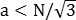⌊ (a + 3)/2 ⌋ + 2 ⌊ N2/(6a) ⌋ + ⌊ [(⌊ N2/a ⌋ mod 6) + 3]/4 ⌋
A list of values of g500(a), Lg500(a) and Ug500(a) is tabulated here.

#### Greater Central Divisor

Let hN(b) denote the number of occurrences of b as the GCD of the entries in the N x N MMT; hN(b) is the number of entries in column b of the MMT. A histogram of h500(b) is shown below, along with upper and lower bounds defined in the following sections: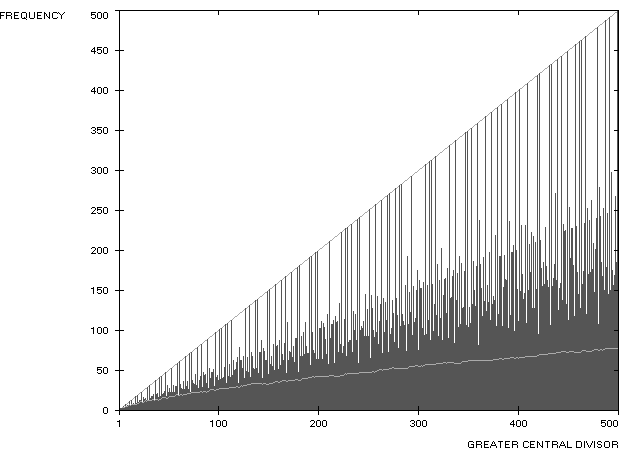Although the GCD frequency distribution has a general appearance similar to the totient function, and a difference of just 1 at prime values of b, nearly all of the other values of the functions differ significantly.

Lower Bound

As can be seen in a previous diagram, central divisors occur for composite column indices C at prime-numbered rows beginning at (C - 1)/2 and extending to the main diagonal:

(C - 1)/2 ≤ prime R ≤ C
or
R > ⌈ (C - 3)/2 ⌉
This result is established in Lemma 7 with C ≤ 2R + 1. By Lemma 13, central divisors occur at every row above the parabola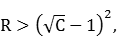or for every R satisfying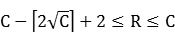Additional central divisors can occur elsewhere in column C, so the preceding ranges form a lower bound for hN(C) that also applies to prime columns. The number of primes up to the parabola is: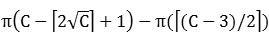and the total number of values above the parabola is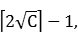so the lower bound is the sum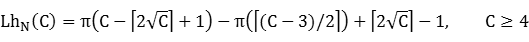It is conjectured that a tighter lower bound for hN(C) is simply π(C).

Upper Bound

Since a prime-numbered column of the MMT is completely filled with central divisors down to the main diagonal, an upper bound for hN(b) is

UhN(b) = b

### Appendix: Mathematical Results

Many of these results involve central divisors.

#### Lemma 1

If a,b,c,d ∈ ℕ such that
 ab = cd (13)
and
 | a - b | = | c - d | (14)
then
a = c and b = d.
It follows that a pair of central divisors are uniquely defined by their product and their unsiqned (or squared) difference. Equivalently, if there are two divisor pairs of a natural number with the same unsigned difference, then they are the same pair.

Proof. Without loss of generality, we can take a ≤ b and c ≤ d. From (13), we have

d = ab/c
Substituting in (14) and squaring,
(a - b)2 = (c - ab/c)2

(c2 - a2)(c2 - b2) = 0

c = a and/or c = b

If c = b, then ab = bd by (13), so d = a. But then the assumption c ≤ d is equivalent to b ≤ a, a contradiction. Therefore c = a, and b = d by (13).

#### Lemma 2

{1,p} are central divisors if and only if p = 1 or p is a prime.

Proof. If p = 1, {1,1} are trivially central divisors. If p is a prime, then {1,p} is the only divisor pair of p and therefore its central divisors. Conversely, if p ≠ 1 is not a prime, then p ≥ 4 and p has a proper divisor k such that

2 ≤ k ≤ p/2

2 ≤ p/k ≤ p/2

Combining these two inequalities, we have
|k - p/k| ≤ p/2 - 2 < p - 1
Therefore, {1,p} cannot be central divisors since they are farther apart than {k,p/k}, QED.

#### Lemma 3

{p,q} are central divisors for any primes p and q.

Proof. The divisor pairs of pq are {1,pq} and {p,q}. The first pair cannot be central divisors by Lemma 2 since pq is composite, so {p,q} are central divisors.

#### Lemma 4

For a,b,c,d ∈ ℕ with ab = cd, {c,d} are farther apart than {a,b}, and therefore are not central divisors, when c and/or d is greater than both a and b. In general, {c,d} are not central divisors if
max{c,d} > max{a,b}
or
min{c,d} < min{a,b}
Proof. Let N = ab, so that b = N/a and d = N/c. The first condition above is equivalent to c > max{a,N/a} and/or N/c > max{a,N/a}. In the first case, N/c < min{a,N/a}, so c and N/c are farther apart than a and N/a. In the second case, c < min{a,N/a}, so again c and N/c are farther apart than a and N/a.

Conversely, the second condition above is equivalent to c < min{a,N/a} and/or N/c < min{a,N/a}. In the first case, N/c > max{a,N/a}, so c and N/c are farther apart than a and N/a. In the second case, c > max{a,N/a}, so again c and N/c are farther apart than a and N/a, QED.

#### Lemma 5

For primes p and q, {max{p,q},pq} are central divisors.

Proof. Without loss of generality, assume p ≥ q so that {max{p,q},pq} = {p,pq}. By Lemma 2, {1,p2q} cannot be central divisors since the latter factor is composite. The remaining factor pairs of p2q are {q,p2} and the reference pair {p,pq}. These pairs are the same if p = q, so assume p > q. Then since

p2 > p and p2 > pq,
p2 is greater than both reference factors, and therefore {q,p2} are not central divisors by Lemma 4. The remaining factor pair {p,pq} = {max{p,q},pq} must be central divisors, QED.

#### Lemma 6

Let w,x,y,z be natural numbers with
 (w - 1)(x - 1) < yz < (w + 1)(x + 1) (15)
Then
 (y - x)(z - w) ≤ 0 (16)
Proof by contradiction. Assume (16) does not hold:
(y - x)(z - w) > 0
Then
y > x and z > w
or
y < x and z < w
In the first case, we have
y ≥ x + 1 and z ≥ w + 1

yz ≥ (x + 1)(w + 1),

contradicting (15). In the second case, we have
y ≤ x - 1 and z ≤ w - 1

yz ≤ (x - 1)(w - 1)

#### Lemma 7

If p is a prime and n is a natural number such that
n ≤ 2p + 1,
then {n,p} are central divisors.

Proof. When n = 1, {n,p} are central divisors by Lemma 2, so we can henceforth assume n ≥ 2. If n is prime, then {n,p} are central divisors by Lemma 3, regardless of the size of n.

Otherwise, let k be any proper divisor of composite n. The divisor pairs of np are {1,np}, {k,pn/k}, {pk,n/k} and the reference pair {n,p}. The first pair cannot be central divisors by Lemma 2 since np is composite.

Next, we have

k ≤ n/2 ≤ p + 1/2
If k = p, then {k,pn/k} is the same as the reference pair, so we can assume k < p. Since k < n, we have
pn/k > n and pn/k > p,
so pn/k is greater than both reference factors, and therefore {k,pn/k} cannot be central divisors by Lemma 4.

Now assume that n = 2p + 1. Since n is odd, k ≥ 3 and

n/p = 2 + 1/p < 3 ≤ k,
or pk > n. If n = 2p, then {n,p} = {p,2p} are central divisors by Lemma 5. Otherwise, we have n ≤ 2p - 1 and
n/p ≤ 2 - 1/p < 2 ≤ k,
so again pk > n. Since
pk ≥ 2p > p,
pk is greater than both reference factors, and so {pk,n/k} cannot be central divisors by Lemma 4, QED.

#### Lemma 8

If {a,b} are central divisors, then so are {m,ab} for all natural numbers m such that
 (a - 1)(b - 1) < m < (a + 1)(b + 1) (17)
If ab is composite, these limits are closer together than for any other factor pair of ab.

Proof. If ab is a prime p, then its central divisors are {1,p} by Lemma 2, 1 ≤ m ≤ 2p + 1 by (17), and {m,p} are central divisors by Lemma 7.

To prove the second part when ab is composite, let {c,d} be another factor pair of ab = cd. Then by the definition of central divisors,

|d - c| > |b - a|

d + c > b + a

(c + 1)(d + 1) > (a + 1)(b + 1)

and similarly,
-(c + d) < -(a + b)

(c - 1)(d - 1) < (a - 1)(b - 1)

Therefore, the limits in (17) are farther apart for {c,d} than for {a,b}.

For {m,ab} to be central divisors, we must show that

 (m - ab)2 ≤ (k - mab/k)2 (18)
for all divisors k of the product mab. Each such divisor can be written as k = ij, where i is a divisor of m and j is a divisor of ab. It follows from the preceding remarks that (17) holds for i and j:
(j - 1)(ab/j - 1) < i(m/i) < (j + 1)(ab/j + 1)
From Lemma 6 with w = j, x = ab/j, y = i and z = m/i, we have
(i - ab/j)(m/i - j) ≤ 0,
which reduces to (18), QED.

#### Lemma 9

If p and q are primes and n is a natural number such that
(p - 1)(q - 1) < n < (p + 1)(q + 1)
then {n,pq} are central divisors.

Proof. {p,q} are central divisors by Lemma 3. The result follows by Lemma 8 with a = p and b = q.

#### Lemma 10

For natural numbers a and b,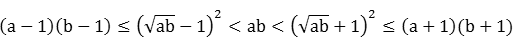Proof. Clearly,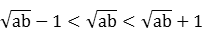We have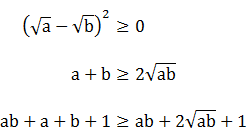(19)
From (19), we also have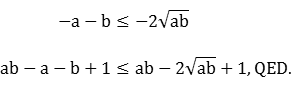#### Lemma 11

Let a and b be natural numbers, with b composite and k a proper divisor of b.
If a < b/k, then {a,b} are not central divisors.
If a > b/k, then {ka,b/k} are not central divisors.
Proof. Since k ≥ 2, we have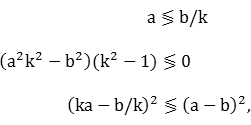so the factor pair {a,b} is farther apart than {ka,b/k}, or conversely.

#### Lemma 12

For primes p, q and r, {p,qr} are central divisors when p > q and p > r.

Proof. The divisor pairs of pqr are {1,pqr}, {q,pr}, {r,pq} and the reference pair {p,qr}. The first cannot be central divisors by Lemma 2 since pqr is composite. We have q < p = pr/r, so {q,pr} cannot be central divisors by Lemma 11 with a = q, b = pr and k = r. Similarly, r < p = pq/q, so {r,pq} cannot be central divisors by Lemma 11 with a = r, b = pq and k = q.

#### Lemma 13

If a and b are natural numbers such that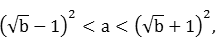(20)
then {a,b} are central divisors. The condition is symmetric in a and b; (20) is equivalent to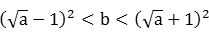(21)
Proof. Assume {a,b} are not the central divisors of ab. Then there exist other natural numbers c and d such that cd = ab and c and d are closer together than a and b. Without loss of generality, we can take a < b and c ≤ d. By assumption,
b - a > d - c ≥ 0

(b - a)2 > (d - c)2

Now 4ab = 4cd, so adding that quantity to both sides, we have
(b + a)2 > (d + c)2

 b + a > d + c (22) d + c ≤ b + a - 1 (23)

Using (21),By (23),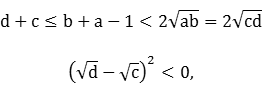a contradiction. Therefore, {a,b} are Central Divisors when inequality (20) holds, QED.

Note that the converse does not hold; for example, if a = 1 and b = 5, then {a,b} are Central Divisors but neither (20) nor (21) hold.

#### Lemma 14

For any natural number n ≥ 2, {n,n - 1}, {n,n}, {n,n + 1}, {n,n + 2} and {n,n + 3} are central divisors.

Proof. Let m ∈ {-1,0,1,2,3}. Then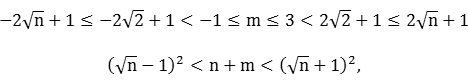so the inequality in Lemma 13 is satisfied for a = n and b = n + m, and therefore {n,n + m} are central divisors, QED. The set of values for m increases in both directions for larger minimum values of n.

#### Lemma 15

If p and q ≤ p + 1 are primes and n is a natural number such that
 p(q - 2) ≤ n ≤ (p - 1)(q - 1), (24)
then {n,pq} are central divisors if and only if n is not divisible by q - 1. In particular, when q = 3, the range for n is
p ≤ n ≤ 2(p - 1)
and {n,3p} are central divisors if and only if n is odd.

Proof. A valid range for n exists only when q ≤ p + 1, and we also have n < pq. Define the following logical statements:

A: n is not divisible by q - 1

B: {n,pq} are central divisors,

so that Lemma 15 is simply A ⇔ B, subject to (24). We will first prove the converse B ⇒ A, or equivalently, ~A ⇒ ~B.

Assume q - 1 | n (A is false). Then by (24),

n/(q - 1) ≤ p - 1 < p

[p(q - 1) - nq/(q - 1)]2 < (pq - n)2,

so {p(q - 1),qn/(q - 1)} are closer together than {n,pq}, and therefore {n,pq} are not central divisors (B is false).

Next, we'll prove some special cases that serve to limit the range of the variables. Note that Lemma 15 is proven when either both A and B are true, or both A and B are false, subject to inequality (24).

##### q = 2
If q = 2, then q - 1 = 1 divides every value of n and A is false. From (24) we have n < p, so {n,2p} are farther apart than {2n,p} and therefore {n,pq} are not central divisors (B is also false).
##### q = p + 1
If q = p + 1, then n = p(p - 1) is divisible by q - 1 = p and A is false. Since npq = p2(p2 - 1) can be written as the product of two consecutive integers, these are its central divisors by Lemma 14, and therefore {n,pq} = {p2 - p,p2 + p} are not, establishing that B is also false. We can now take p ≥ q ≥ 3.
##### p = q
From (24), we have n = p(p - 2) = (p - 1)2 - 1 or n = (p - 1)2. In the former case, p - 1 does not divide n since it would leave a remainder, so A is true. Then {n,pq} = {p(p - 2),p2} are central divisors since they are closer together than the other factor pairs of npq:
{1,p3(p - 2)} are not central divisors by Lemma 2 since npq is composite.

{p,p2(p - 2)} are not central divisors since they are farther apart than {n,pq} for p > 3. If p = 3, these pairs are the same.

{p - 2,p3} are not central divisors since they are farther apart than {n,pq}

Therefore, B is also true.

In the latter case, p - 1 divides n so A is false. Then {n,pq} = {(p - 1)2,p2} are not central divisors since they are farther apart than {p(p - 1),p(p - 1)}, so B is also false.

##### q = 3 and n = p
In this case, n is not divisible by q - 1 = 2 since p ≥ 3, and therefore A is true. Then {n,pq} = {p,3p} are central divisors by Lemma 5, so B is also true. For any larger value of q, the left side of (24) is greater than p, so we can henceforth take
 n > p > q ≥ 3
##### Prime n
If n is prime, then it is not divisible by q - 1 ≥ 2 and so A is true. We can exclude the composite endpoints in inequality (24):
p(q - 2) < n < (p - 1)(q - 1)
It follows that n > q, so {n,pq} are central divisors by Lemma 12, and B is true.
##### Composite n
Lastly, when n is composite, it has a proper divisor k such that 2 ≤ k ≤ n/2. If {n,pq} are not central divisors (B is false), then they are some other divisor pair of npq:
{1,npq} are not central divisors by Lemma 2 since npq is composite.

{p,nq} are not central divisors since they are farther apart than {n,pq}.

{q,np} are not central divisors since they are farther apart than {n,pq}.

{k,pqn/k} are not central divisors since they are farther apart than {n,pq}.

The remaining factor pairs of npq are of the form {kp,qn/k} or {kq,pn/k} for k ≤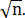Grouping the smaller (or larger) factors together increases the distance between the corresponding products, so {kq,pn/k} are not central divisors since they are farther apart than {kp,qn/k}.

Therefore, {kp,qn/k} are the central divisors of npq and so must be closer together than {n,pq}. Then
n/p < k < q
But by (24), n/p ≥ q - 2, so k > q - 2 and k ≤ q - 1. The only possibility is k = q - 1, so q - 1 divides n and A is false. This proves ~B ⇒ ~A, or equivalently, A ⇒ B, QED.

#### Lemma 16

If p is a prime and n < p is a natural number, then n and p - n are coprime.

Proof. Since p is prime and n < p, n and p cannot have any common factors other than 1, so gcd(n,p) = 1. By , gcd(n,p-n) = gcd(n,p-n+n) = gcd(n,p), QED.

#### Lemma 17

For a natural number n and all composite numbers c > n2, {n,c} are not central divisors.

Proof. Since c is composite, it has a factor k between 2 and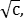inclusive. We have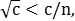so

k < c/n

(n2k2 - c2)(k2 - 1) < 0

(nk - c/k)2 < (n - c)2

and therefore {nk,c/k} are closer together than {n,c}, QED.

#### Lemma 18

For integers i, j, k, m and n such that 0 ≤ m < k ≤ i ≤ j, the quantity of values of n such that i ≤ n ≤ j and n mod k = m is
⌊ (j - m)/k ⌋ - ⌈ (i - m)/k ⌉ + 1
Proof. Due to the requirement that n mod k = m, the values to be counted are at intervals of k. Since n = k ⌊ n/k ⌋ + m, we write
i - m ≤ k ⌊ n/k ⌋ ≤ j - m
and consider only multiples of k in the interval. The smallest multiple of k that is at least as large as the lower bound is k⌈ (i - m)/k ⌉ and the largest multiple of k not exceeding the upper bound is k ⌊ (j - m)/k ⌋, so
k⌈ (i - m)/k ⌉ ≤ k ⌊ n/k ⌋ ≤ k ⌊ (j - m)/k ⌋
Dividing by k,
⌈ (i - m)/k ⌉ ≤ ⌊ n/k ⌋ ≤ ⌊ (j - m)/k ⌋
and the result follows.

#### Lemma 19

If m is the smallest proper divisor of a composite natural number n, p is a prime, and n ≤ mp, then {n,p} are central divisors.

Proof. Since m is the smallest proper divisor of n, n/m is its largest, so for all proper divisors k of n, we have

m ≤ k ≤ n/m ≤ p
The divisor pairs of np are {1,np}, {kp,n/k}, {k,pn/k} and the reference pair {n,p}. {1,np} cannot be central divisors by Lemma 2 since np is composite.

If p | n, then n/p ≤ m; otherwise, n/p < m ≤ k. In the former case, we can exclude k = n/p when comparing {kp,n/k} with {n,p} since that would result in both pairs being identical. Therefore, k > n/p, so kp > n and kp > p. Since kp is greater than both reference factors, {kp,n/k} cannot be central divisors by Lemma 4.

Finally, since k < kp and pn/k > n/k, {k,pn/k} are farther apart than {kp,n/k} and also cannot be central divisors, QED.

#### Lemma 20

{n,p} are central divisors for prime p and odd natural numbers n such that n ≤ 3p.

Proof. If n is prime, then {n,p} are central divisors by Lemma 3. Otherwise, n is composite and its smallest proper divisor is at least 3 since it is odd. The result follows from Lemma 19 with m = 3.

#### Lemma 21

{n,p} are central divisors for prime p and natural numbers n such that n ≤ 5p and n mod 6 = ±1.

Proof. If n is prime, then {n,p} are central divisors by Lemma 3. Otherwise, n is composite and of the form 6i ± 1 for some natural number i. Dividing n by 2 or 3 leaves a fractional remainder, so the smallest proper divisor of n is 5. The result follows from Lemma 19 with m = 5.

#### Lemma 22

For a prime p and a natural number n, {pn,pn+1} are central divisors.

Proof. By Lemma 2, {1,p2n+1} cannot be central divisors since the latter factor is composite. The remaining factor pairs of p2n+1 are {pk,p2n+1-k} for k = 1,...,n-1, and the reference pair {pn,pn+1}. Since k < n, we have

2n + 1 - k > n + 1

p2n+1-k > pn+1 > pn,

so p2n+1-k is greater than both reference factors for all given values of k, and therefore {pk,p2n+1-k} are not central divisors by Lemma 4, QED.

#### Lemma 23

If {a,b} are central divisors and d | gcd(a,b), then {a/d,b/d} are also central divisors. For example, {18,20} are central divisors of 360 and gcd(18,20) = 2, so {9,10} are also central divisors.

Proof. Let N = ab. Since {a,b} are the central divisors of N, then a minimizes (a - N/a)2 over all divisors of N. Let d | gcd(a,b); that is, d | a and d | b. Then

(a - N/a)2/d2 = (a/d - (N/a)/d)2
is also minimized for all such d. Therefore, {a/d,b/d} are central divisors of N/d2.

#### Lemma 24

For a, b, n ∈ ℕ, if a < n < b or n < b/a or n < a/b, then {na,nb} are not central divisors.

Proof. In the first case, we have

(n - a)(n - b) < 0

(n2 - a2)(n2 - b2) < 0

(n2 - ab)2 < (na - nb)2,

so {n2,ab} are closer together than {na,nb}. In the second case, we have
na < b

(n4 - n2)a2 < (n2 - 1)b2

(n2a - b)2 < (na - nb)2,

so {n2a,b} are closer together than {na,nb}. In the last case, we can exchange a and b in the preceding results to establish that {n2b,a} are closer together than {na,nb}, QED.

#### Lemma 25

Central divisors have the minimum arithmetic mean amongst all factor pairs with the same geometric mean.

Proof. Let {a,N/a} be the central divisors of N, and {b,N/b} a different factor pair of the same product,. Then the geometric mean of both factor pairs is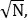and

b < a ≤ N/a < N/b
By (22) of Lemma 13,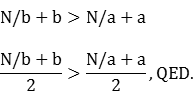#### Lemma 26

For a prime q and natural numbers n and m such that
q - 1 ≤ n ≤ q + 1
and
 q - 2n ≤ m ≤ 3q - 2n + 2, (25)
{qp,np + m} are central divisors for all primes p.

For example, when q = 5,

{5p,4p - 3}, {5p,4p - 2}, ..., {5p,4p + 9},
{5p,5p - 5}, {5p,5p - 4}, ..., {5p,5p + 7},
{5p,6p - 7}, {5p,6p - 6}, ..., {5p,6p + 5}
are each central divisors for all primes p.

The ranges for np + m are disjoint when p > 2q + 4, in which case there are 6q + 9 pairs of central divisors for each value of q.

Proof. Multiplying the first inequality by p - 2 and adding the second inequality plus 2n, we have for all primes p:

(p - 2)(q - 1) + (q - 2n) + 2n ≤ (p - 2)n + m + 2n ≤ (p - 2)(q + 1) + (3q - 2n + 2) + 2n

(p - 1)(q - 1) < np + m < (p + 1)(q + 1)

Since {q,p} are central divisors by Lemma 3, the conditions of Lemma 8 are satisifed and {qp,np + m} are central divisors for all primes p.

To find the condition for which the ranges of central divisors are disjoint, we substitute each value of n in (25):

If n = q - 1:
q - 2(q - 1) ≤ m ≤ 3q - 2(q - 1) + 2

(q - 1)p - q + 2 ≤ np + m ≤ (q - 1)p + q + 4

If n = q:
q - 2q ≤ m ≤ 3q - 2q + 2

qp - q ≤ np + m ≤ qp + q + 2

If n = q + 1:
q - 2(q + 1) ≤ m ≤ 3q - 2(q + 1) + 2

(q + 1)p - q - 2 ≤ np + m ≤ (q + 1)p + q

The first two ranges are disjoint when
(q - 1)p + q + 4 < qp - q

p > 2q + 4

Similarly, the last two ranges are disjoint when
qp + q + 2 < (q + 1)p - q - 2

p > 2q + 4

#### Lemma 27

If a and b are each natural numbers greater than 1, and p is a prime such that
p > (a - 1)(b - 1),
then {p,ab} are central divisors.

Proof. We have

(a - 2)(b - 2) ≥ 0

2p > 2(a - 1)(b - 1) ≥ ab - 2

ab ≤ 2p + 1,

so {ab,p} are central divisors by Lemma 7, QED.

#### Hyperbolas in the multiplication table

If the products in the multiplication table are color-coded according to their magnitude, hyberbolas of the form
RC = constant
produce a rainbow effect:This image is included simply for its visual impact.

### References & Acknowledgements

 P. Erdős, Some remarks on number theory, Riveon Lematematika 9 (1955) 45-48, cited in D. Koukoulopoulos and J. Thiel, Arrangements of stars on the American flag, The American Mathematical Monthly 119, No. 6 (2012), p. 447; available at https://www.maa.org/sites/default/files/pdf/pubs/monthly_june12-stars.pdf

 P. Erdős, An asymptotic inequality in the theory of numbers (in Russian), Vestnik Leningrad. Univ., v. 15 (1960) p. 13, 41-49; MR0126424 (23 #A3720), cited in Finch, S. (2018), Mathematical Constants II (Encyclopedia of Mathematics and Its Applications), p. 33. Cambridge: Cambridge University Press. doi:10.1017/9781316997741, available from OEIS

 K. Ford, The distribution of integers with a divisor in a given interval, Ann. of Math. 168 no. 2 (2008) pp. 367-433, cited in Koukoulopoulos and Thiel, op. cit., p. 448.

 Weisstein, Eric W. "Relatively Prime." From MathWorld--A Wolfram Web Resource. https://mathworld.wolfram.com/RelativelyPrime.html

 Wikipedia Coprime integers

 M. Abramowitz & I. A. Stegun (eds.), Handbook of Mathematical Functions, National Bureau of Standards, 1964, p. 826.

 R. Sedgewick, Algorithms, second ed., Addison-Wesley, 1988, p. 12.

 Wikipedia Greatest common divisor

Thanks to Nigel Boston, Harold Diamond, Martin Huxley and Daniel Fischer for constructive feedback.

This paper is a revision and expansion of a paper first posted on this site in 1993.

MathSite